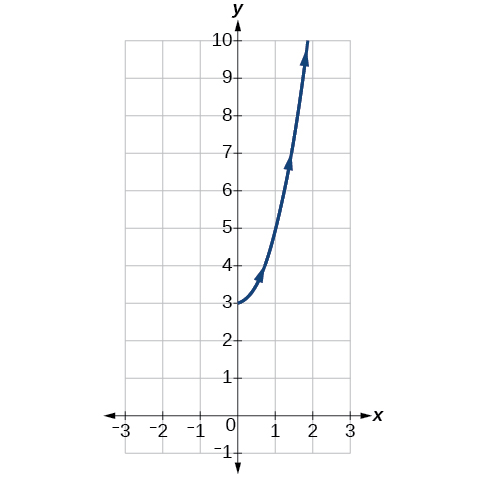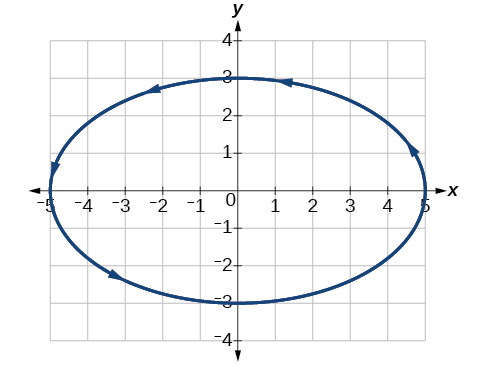# 10.7 Parametric equations: graphs

 Page 1 / 4
In this section you will:
• Graph plane curves described by parametric equations by plotting points.
• Graph parametric equations.

It is the bottom of the ninth inning, with two outs and two men on base. The home team is losing by two runs. The batter swings and hits the baseball at 140 feet per second and at an angle of approximately $\text{\hspace{0.17em}}45°\text{\hspace{0.17em}}$ to the horizontal. How far will the ball travel? Will it clear the fence for a game-winning home run? The outcome may depend partly on other factors (for example, the wind), but mathematicians can model the path of a projectile and predict approximately how far it will travel using parametric equations . In this section, we’ll discuss parametric equations and some common applications, such as projectile motion problems.Parametric equations can model the path of a projectile. (credit: Paul Kreher, Flickr)

## Graphing parametric equations by plotting points

In lieu of a graphing calculator or a computer graphing program, plotting points to represent the graph of an equation is the standard method. As long as we are careful in calculating the values, point-plotting is highly dependable.

Given a pair of parametric equations, sketch a graph by plotting points.

1. Construct a table with three columns: $\text{\hspace{0.17em}}t,x\left(t\right),\text{and}\text{\hspace{0.17em}}\text{\hspace{0.17em}}y\left(t\right).$
2. Evaluate $x$ and $y$ for values of $t$ over the interval for which the functions are defined.
3. Plot the resulting pairs $\text{\hspace{0.17em}}\left(x,y\right).$

## Sketching the graph of a pair of parametric equations by plotting points

Sketch the graph of the parametric equations $x\left(t\right)={t}^{2}+1,\text{\hspace{0.17em}}\text{\hspace{0.17em}}y\left(t\right)=2+t.$

Construct a table of values for $\text{\hspace{0.17em}}t,x\left(t\right),\text{\hspace{0.17em}}$ and $\text{\hspace{0.17em}}y\left(t\right),\text{\hspace{0.17em}}$ as in [link] , and plot the points in a plane.

$t$ $x\left(t\right)={t}^{2}+1$ $y\left(t\right)=2+t$
$-5$ $26$ $-3$
$-4$ $17$ $-2$
$-3$ $10$ $-1$
$-2$ $5$ $0$
$-1$ $2$ $1$
$0$ $1$ $2$
$1$ $2$ $3$
$2$ $5$ $4$
$3$ $10$ $5$
$4$ $17$ $6$
$5$ $26$ $7$

The graph is a parabola with vertex at the point $\text{\hspace{0.17em}}\left(1,2\right),$ opening to the right. See [link] .

Sketch the graph of the parametric equations $\text{\hspace{0.17em}}x=\sqrt{t},\text{\hspace{0.17em}}\text{\hspace{0.17em}}y=2t+3,\text{\hspace{0.17em}}\text{\hspace{0.17em}}\text{\hspace{0.17em}}0\le t\le 3.$## Sketching the graph of trigonometric parametric equations

Construct a table of values for the given parametric equations and sketch the graph:

$\begin{array}{l}\\ \begin{array}{l}x=2\mathrm{cos}\text{\hspace{0.17em}}t\hfill \\ y=4\mathrm{sin}\text{\hspace{0.17em}}t\hfill \end{array}\end{array}$

Construct a table like that in [link] using angle measure in radians as inputs for $\text{\hspace{0.17em}}t,\text{\hspace{0.17em}}$ and evaluating $\text{\hspace{0.17em}}x\text{\hspace{0.17em}}$ and $\text{\hspace{0.17em}}y.\text{\hspace{0.17em}}$ Using angles with known sine and cosine values for $\text{\hspace{0.17em}}t\text{\hspace{0.17em}}$ makes calculations easier.

$t$ $x=2\mathrm{cos}\text{\hspace{0.17em}}t$ $y=4\mathrm{sin}\text{\hspace{0.17em}}t$
0 $x=2\mathrm{cos}\left(0\right)=2$ $y=4\mathrm{sin}\left(0\right)=0$
$\frac{\pi }{6}$ $x=2\mathrm{cos}\left(\frac{\pi }{6}\right)=\sqrt{3}$ $y=4\mathrm{sin}\left(\frac{\pi }{6}\right)=2$
$\frac{\pi }{3}$ $x=2\mathrm{cos}\left(\frac{\pi }{3}\right)=1$ $y=4\mathrm{sin}\left(\frac{\pi }{3}\right)=2\sqrt{3}$
$\frac{\pi }{2}$ $x=2\mathrm{cos}\left(\frac{\pi }{2}\right)=0$ $y=4\mathrm{sin}\left(\frac{\pi }{2}\right)=4$
$\frac{2\pi }{3}$ $x=2\mathrm{cos}\left(\frac{2\pi }{3}\right)=-1$ $y=4\mathrm{sin}\left(\frac{2\pi }{3}\right)=2\sqrt{3}$
$\frac{5\pi }{6}$ $x=2\mathrm{cos}\left(\frac{5\pi }{6}\right)=-\sqrt{3}$ $y=4\mathrm{sin}\left(\frac{5\pi }{6}\right)=2$
$\pi$ $x=2\mathrm{cos}\left(\pi \right)=-2$ $y=4\mathrm{sin}\left(\pi \right)=0$
$\frac{7\pi }{6}$ $x=2\mathrm{cos}\left(\frac{7\pi }{6}\right)=-\sqrt{3}$ $y=4\mathrm{sin}\left(\frac{7\pi }{6}\right)=-2$
$\frac{4\pi }{3}$ $x=2\mathrm{cos}\left(\frac{4\pi }{3}\right)=-1$ $y=4\mathrm{sin}\left(\frac{4\pi }{3}\right)=-2\sqrt{3}$
$\frac{3\pi }{2}$ $x=2\mathrm{cos}\left(\frac{3\pi }{2}\right)=0$ $y=4\mathrm{sin}\left(\frac{3\pi }{2}\right)=-4$
$\frac{5\pi }{3}$ $x=2\mathrm{cos}\left(\frac{5\pi }{3}\right)=1$ $y=4\mathrm{sin}\left(\frac{5\pi }{3}\right)=-2\sqrt{3}$
$\frac{11\pi }{6}$ $x=2\mathrm{cos}\left(\frac{11\pi }{6}\right)=\sqrt{3}$ $y=4\mathrm{sin}\left(\frac{11\pi }{6}\right)=-2$
$2\pi$ $x=2\mathrm{cos}\left(2\pi \right)=2$ $y=4\mathrm{sin}\left(2\pi \right)=0$

[link] shows the graph.

By the symmetry shown in the values of $x$ and $\text{\hspace{0.17em}}y,\text{\hspace{0.17em}}$ we see that the parametric equations represent an ellipse . The ellipse is mapped in a counterclockwise direction as shown by the arrows indicating increasing $\text{\hspace{0.17em}}t\text{\hspace{0.17em}}$ values.

Graph the parametric equations: $\text{\hspace{0.17em}}x=5\mathrm{cos}\text{\hspace{0.17em}}t,\text{\hspace{0.17em}}\text{\hspace{0.17em}}y=3\mathrm{sin}\text{\hspace{0.17em}}t.$## Graphing parametric equations and rectangular form together

Graph the parametric equations $\text{\hspace{0.17em}}x=5\mathrm{cos}\text{\hspace{0.17em}}t\text{\hspace{0.17em}}$ and $\text{\hspace{0.17em}}y=2\mathrm{sin}\text{\hspace{0.17em}}t.\text{\hspace{0.17em}}$ First, construct the graph using data points generated from the parametric form . Then graph the rectangular form of the equation. Compare the two graphs.

Construct a table of values like that in [link] .

$t$ $x=5\mathrm{cos}\text{\hspace{0.17em}}t$ $y=2\mathrm{sin}\text{\hspace{0.17em}}t$
$\text{0}$ $x=5\mathrm{cos}\left(0\right)=5$ $y=2\mathrm{sin}\left(0\right)=0$
$\text{1}$ $x=5\mathrm{cos}\left(1\right)\approx 2.7$ $y=2\mathrm{sin}\left(1\right)\approx 1.7$
$\text{2}$ $x=5\mathrm{cos}\left(2\right)\approx -2.1$ $y=2\mathrm{sin}\left(2\right)\approx 1.8$
$\text{3}$ $x=5\mathrm{cos}\left(3\right)\approx -4.95$ $y=2\mathrm{sin}\left(3\right)\approx 0.28$
$\text{4}$ $x=5\mathrm{cos}\left(4\right)\approx -3.3$ $y=2\mathrm{sin}\left(4\right)\approx -1.5$
$\text{5}$ $x=5\mathrm{cos}\left(5\right)\approx 1.4$ $y=2\mathrm{sin}\left(5\right)\approx -1.9$
$-1$ $x=5\mathrm{cos}\left(-1\right)\approx 2.7$ $y=2\mathrm{sin}\left(-1\right)\approx -1.7$
$-2$ $x=5\mathrm{cos}\left(-2\right)\approx -2.1$ $y=2\mathrm{sin}\left(-2\right)\approx -1.8$
$-3$ $x=5\mathrm{cos}\left(-3\right)\approx -4.95$ $y=2\mathrm{sin}\left(-3\right)\approx -0.28$
$-4$ $x=5\mathrm{cos}\left(-4\right)\approx -3.3$ $y=2\mathrm{sin}\left(-4\right)\approx 1.5$
$-5$ $x=5\mathrm{cos}\left(-5\right)\approx 1.4$ $y=2\mathrm{sin}\left(-5\right)\approx 1.9$

Plot the $\text{\hspace{0.17em}}\left(x,y\right)\text{\hspace{0.17em}}$ values from the table. See [link] .

Next, translate the parametric equations to rectangular form. To do this, we solve for $\text{\hspace{0.17em}}t\text{\hspace{0.17em}}$ in either $\text{\hspace{0.17em}}x\left(t\right)\text{\hspace{0.17em}}$ or $\text{\hspace{0.17em}}y\left(t\right),\text{\hspace{0.17em}}$ and then substitute the expression for $\text{\hspace{0.17em}}t\text{\hspace{0.17em}}$ in the other equation. The result will be a function $y\left(x\right)$ if solving for $\text{\hspace{0.17em}}t\text{\hspace{0.17em}}$ as a function of $\text{\hspace{0.17em}}x,\text{\hspace{0.17em}}$ or $\text{\hspace{0.17em}}x\left(y\right)$ if solving for $\text{\hspace{0.17em}}t\text{\hspace{0.17em}}$ as a function of $\text{\hspace{0.17em}}y.\text{\hspace{0.17em}}$

Then, use the Pythagorean Theorem    .

$\begin{array}{r}\hfill {\mathrm{cos}}^{2}t+{\mathrm{sin}}^{2}t=1\\ \hfill {\left(\frac{x}{5}\right)}^{2}+{\left(\frac{y}{2}\right)}^{2}=1\\ \hfill \frac{{x}^{2}}{25}+\frac{{y}^{2}}{4}=1\end{array}$

#### Questions & Answers

x exposant 4 + 4 x exposant 3 + 8 exposant 2 + 4 x + 1 = 0
x exposent4+4x exposent3+8x exposent2+4x+1=0
HERVE
How can I solve for a domain and a codomains in a given function?
ranges
EDWIN
Thank you I mean range sir.
Oliver
proof for set theory
don't you know?
Inkoom
find to nearest one decimal place of centimeter the length of an arc of circle of radius length 12.5cm and subtending of centeral angle 1.6rad
factoring polynomial
find general solution of the Tanx=-1/root3,secx=2/root3
find general solution of the following equation
Nani
the value of 2 sin square 60 Cos 60
0.75
Lynne
0.75
Inkoom
when can I use sin, cos tan in a giving question
depending on the question
Nicholas
I am a carpenter and I have to cut and assemble a conventional roof line for a new home. The dimensions are: width 30'6" length 40'6". I want a 6 and 12 pitch. The roof is a full hip construction. Give me the L,W and height of rafters for the hip, hip jacks also the length of common jacks.
John
I want to learn the calculations
where can I get indices
I need matrices
Nasasira
hi
Raihany
Hi
Solomon
need help
Raihany
maybe provide us videos
Nasasira
Raihany
Hello
Cromwell
a
Amie
What do you mean by a
Cromwell
nothing. I accidentally press it
Amie
you guys know any app with matrices?
Khay
Ok
Cromwell
Solve the x? x=18+(24-3)=72
x-39=72 x=111
Suraj
Solve the formula for the indicated variable P=b+4a+2c, for b
Need help with this question please
b=-4ac-2c+P
Denisse
b=p-4a-2c
Suddhen
b= p - 4a - 2c
Snr
p=2(2a+C)+b
Suraj
b=p-2(2a+c)
Tapiwa
P=4a+b+2C
COLEMAN
b=P-4a-2c
COLEMAN
like Deadra, show me the step by step order of operation to alive for b
John
A laser rangefinder is locked on a comet approaching Earth. The distance g(x), in kilometers, of the comet after x days, for x in the interval 0 to 30 days, is given by g(x)=250,000csc(π30x). Graph g(x) on the interval [0, 35]. Evaluate g(5)  and interpret the information. What is the minimum distance between the comet and Earth? When does this occur? To which constant in the equation does this correspond? Find and discuss the meaning of any vertical asymptotes.
The sequence is {1,-1,1-1.....} hasByByByBy David Martin